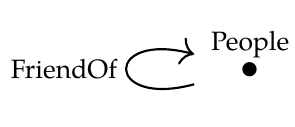Anindya wrote:

> K, let's take \$$\mathcal{C}\$$ to be the people/friend-of schema from [Lecture 42](https://forum.azimuthproject.org/discussion/2236/lecture-42-chapter-3-transforming-databases).

>> And \$$\mathcal{D}\$$ is the same category but without the friend-of arrow. There's only one choice for \$$G : \mathcal{D} \rightarrow \mathcal{C}\$$, which is the obvious inclusion functor.

Yes, that's the example I had in mind! \$$\mathrm{Lan}_G\$$ generates an infinite list of friends for any person, so if start with a database \$$H\$$ with just one person, then form \$$\mathrm{Lan}_G(H)\$$, then form \$$\mathrm{Lan}_G(H) \circ G\$$, we've got an enormous database with that person and an infinite list of others.

> I'm guessing that the issue here is that the inclusion functor \$$G\$$ is not full.

That sounds about right. It would be fun to work out necessary and sufficient conditions.